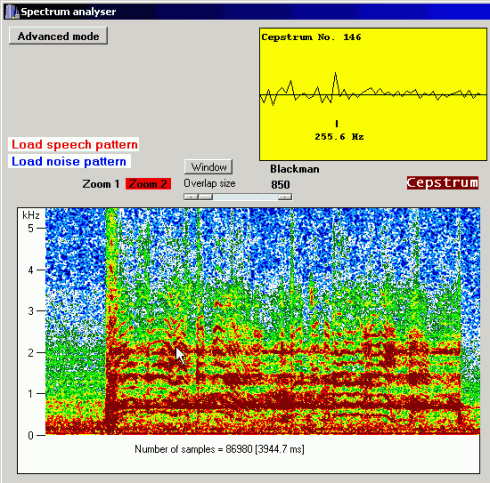Multitask Noisy Speech Enhancement System

www.denoise.net

Overview
Example
Restorer
- Speech band equalizer
- Dynamics processing
- Noise gate
- Signal level limiter
- Clipping restoration
- Noise reduction
- Noise whitening
- Blind deconvolution
- Spectrum analyser
- Time stretching
- Spectral expander
- Fourier corrector
- Neural network corrector
- Decorrelation
- Joint approximation
- Homomorphic approximation
- Reverberation
Recorder
Browser
- Synchronisation
- Normalisation
Contact info

# Spectrum analyser

The spectrum analyser module computes:

• instantaneous spectrum of the signal;
• average spectrum;
• instantaneous power cepstrum (the Fourier transform of the log-magnitude spectrum), useful to determine the changes in frequency of the glottal tone;
• cepstrally-smoothed instantaneous spectrum, useful to determine the order of the homomorphic analysis.

The module presents the signal spectrum as a full-color sonogram (plot of spectrum magnitude vs. time and frequency). The plot is useful in selecting part of the recording and saving it as a noise or speech pattern to a file for further processing (e.g. using the Fourier corrector or spectral expander modules). The maximum length of the plotted signal is 11 seconds, maximum frequency: 5.5 kHz, minimum time resolution: 23.22 ms, minimum frequency resolution: 21.53 Hz. Spectrum magnitude is presented as a colour selected from the palette (10 colours in the palette). Maximum magnitude and colour contrast may be adjusted by the user to match the colour palette to the dynamic range of the signal.

The analysed signal is divided into segments, 1024 samples each. The segments are overlapping, the overlapping length may be selected by the user or automatically by the algorithm. The second plot presents instantaneous spectrum, power cepstrum or the smoothed spectrum (depending on the option selected in the application) of the segment determined by the position of the cursor in the sonogram.

Instantaneous spectrum

The instantaneous spectrum is computed in the segment selected by the cursor in the sonogram and presented in the second plot. The spectrum analysis algorithm uses short time Fourier transform (STFT). A Fourier transform is computed for each segment: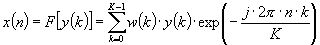x(n) - complex spectrum,
y(k) - analysed segment
w(k) - time window
K - segment length (K=1024)

One of five time windows may be used in analysis (rectangular, Bartlett, Hanning, Hamming, Blackman).

Average spectrum

The average spectrum of the signal is computed using the spectrum analysis of the whole recording or its selected part. If the instantaneous spectrum of the i-th signal segment is: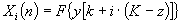then the average signal spectrum is: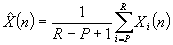y[n] - analysed signal
i - segment number
K - segment length (1024 samples)
P - number of the first segment
R - number of the last segment

The average spectrum plot is useful if the user needs to determine frequency bands where noise and distortions are present. Additionally, using this plot, parts of the recording may be classified as 'noise' or 'signal' and saved to a file as a pattern. To plot average spectrum, the user needs to select the part of recording that will be used in computation, in the sonogram.

Power cepstrum

The power cepstrum of the signal is computed using the cosine transform in the segment selected by the cursor in the sonogram and presented in the second plot. Frequency range up to ca. 2200 Hz is analysed (almost all energy related to voice phonemes is situated in this frequency range). Power cepstrum is computed using the instantaneous spectrum of the selected signal segment: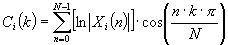k - cepstral coefficient.

The computed cepstrum is plotted. Additionally, time position in the recording and the glottal tone frequency (if found) are displayed. Glottal tone frequency is estimated using a local cepstrum maximum and second-order interpolation. Cepstrum analysis results may be used in time stretching and homomorphic approximation modules.

Cepstral smoothing

The spectrum of the signal computed using the previously described algorithm may be cepstrally smoothed: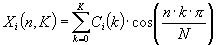K - smoothing order.

Cepstral smoothing restores the time envelope of the speech signal. The smoothed spectrum of the signal is computed in the segment selected by the cursor in the sonogram and presented in the second plot.